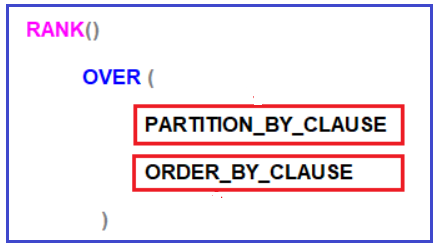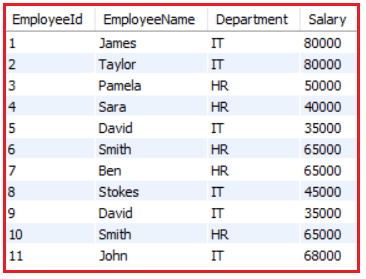# Rank Function in MySQL

## Rank Function in MySQL with Examples

In this article, I am going to discuss Rank Function in MySQL with Examples. Please read our previous article where we discussed Row_Number Function in MySQL. At the end of this article, you will understand what exactly the Rank function is and when and how to use the Rank function in MySQL with real-time examples.

##### RANK Function in MySQL

The MySQL RANK Function is used to return sequential numbers starting from 1 based on the ordering of rows imposed by the ORDER BY clause. When you have two records with the same data, then it will give the same rank to both the rows.

The following is the syntax to use the RANK function in MySQL. As you can see, like the Row_Number function, here also both the Partition by and Order by Clause are optional.The PARTITION BY clause is used to partition the result set into multiple groups. As it is optional, if you did not specify the PARTITION BY clause, then the RANK function will treat the entire result set as a single partition. The ORDER BY clause is also optional and this clause is used to define the sequence in which each row is going to assign their RANK i.e. number.

Note: The RANK function in MySQL returns an increasing unique number for each row starting from 1 and the same for each partition. When there are duplicates or ties, the same rank will be assigned to all the duplicate rows or tie rows, but the next row after the duplicate or tie rows will have the rank it would have been assigned if there had been no duplicates. So, the RANK function skips rankings if there are duplicates or ties.

##### Examples to understand Rank function in MySQL:

We are going to use the following Employees table to understand the MySQL RANK function.Please use the below SQL Script to create the database and Employees table as well as populate the Employees table with the sample data.

```CREATE DATABASE EmployeeDB;
USE EmployeeDB;

CREATE TABLE Employees
(
EmployeeId INT PRIMARY KEY,
EmployeeName VARCHAR(50),
Department VARCHAR(10),
Salary INT
);

Insert Into Employees Values (1, 'James', 'IT', 80000);
Insert Into Employees Values (2, 'Taylor', 'IT', 80000);
Insert Into Employees Values (3, 'Pamela', 'HR', 50000);
Insert Into Employees Values (4, 'Sara', 'HR', 40000);
Insert Into Employees Values (5, 'David', 'IT', 35000);
Insert Into Employees Values (6, 'Smith', 'HR', 65000);
Insert Into Employees Values (7, 'Ben', 'HR', 65000);
Insert Into Employees Values (8, 'Stokes', 'IT', 45000);
Insert Into Employees Values (9, 'David', 'IT', 35000);
Insert Into Employees Values (10, 'Smith', 'HR', 65000);
Insert Into Employees Values (11, 'John', 'IT', 68000);
```
##### MySQL RANK Function without PARTITION BY Clause

Let us first see an example of the MySQL RANK function without using the PARTITION BY Clause. When we did not specify the PARTITION BY Clause, then the RANK function will treat the entire result set as a single partition and give consecutive numbering starting from 1 except when there is a tie.

The following is an example of the MySQL RANK function without using the PARTITION BY clause. Here we use the Order by Clause on the Name column. So, it will give the rank based on the Name column.

```SELECT EmployeeId, Department, Salary, EmployeeName,
RANK() OVER (ORDER BY EmployeeName ASC ) AS 'Rank'
FROM Employees;
```

Once you execute the above SQL query, you will get the following output. As you can see in the below output, there will be no partition and hence all the rows are assigned with consecutive integer numbers starting from 1 except when there is a tie i.e. for the Employee name David, and Smith, it gives the same rank to both the rows.The Rank function in MySQL skips the ranking(s) when there is a tie. As you can see in the above output, Rank 3, and 9 are skipped as there are 2 rows at rank 2 as well as 2 rows at rank 8. The fourth row gets rank 4 and the 10th row gets rank 10.

##### MySQL RANK Function with PARTITION BY clause:

Now, let us see an example of the MySQL RANK function using the PARTITION BY clause. When we specify the PARTITION BY Clause, then the result set is partitioned based on the column which we specify in the Partition BY clause.

Please have a look at the following example code. As you can see, we have specified Department in the Partition By clause and Salary in the Order By clause. As in our Employees table, we have two departments (IT and HR). So, the Partition by Clause will divide the result set into two partitions. One partition is for IT department employees and the other partition is for the HR department employees. Then on each partition, the data is sorted based on the Salary column. The MySQL RANK function then gives an integer sequence number starting from 1 to each record in each partition except when there is a tie. In the case of a tie, it gives the same rank and then skips the ranking.

```SELECT EmployeeId, Department, Salary, EmployeeName,
RANK() OVER (PARTITION BY Department ORDER BY Salary DESC ) AS 'Rank'
FROM Employees;
```

Now, when you execute the above code, you will get the following output.##### Real-time Examples of RANK Functions in MySQL

If you are attending any interview, then one famous question is being asked in almost all interviews i.e. find the nth highest salary. The RANK functions can be used to find the nth highest salary. Let us understand this with an example.

Suppose, there are 2 employees with the FIRST highest salary, then there might be 2 business scenarios as follows:

1. If your business requirement is not to produce any result for the SECOND highest salary then you have to use the MySQL RANK function.
2. If your business requirement is to return the next salary after the tied rows as the SECOND highest Salary, then you have to use the MySQL DENSE_RANK function and we will discuss the DENSE_RANK function in our next article.
###### Fetch the 2nd Highest Salary using the RANK function:

In our Employees table, we have 2 employees with the FIRST highest salary (80000), the Rank() function will not return any data for the SECOND highest Salary. Please execute the below SQL Script and see the output.

```WITH EmployeeCTE  AS
(
SELECT Salary,
RANK() OVER (ORDER BY Salary DESC) AS Rank_Salry
FROM Employees
)
SELECT Salary FROM EmployeeCTE WHERE Rank_Salry = 2 LIMIT 1;
```
###### Fetch the 3rd Highest Salary using the RANK function:

The following example will return the third-highest salary.

```WITH EmployeeCTE  AS
(
SELECT Salary,
RANK() OVER (ORDER BY Salary DESC) AS Rank_Salry
FROM Employees
)
SELECT Salary FROM EmployeeCTE WHERE Rank_Salry = 3 LIMIT 1;
```

Output: 68000

In the next article, I am going to discuss the DENSE_RANK function in MySQL with examples. Here, in this article, I try to explain the need and use of the RANK Function in MySQL with Examples. I hope you enjoy this MySQL RANK Function article.